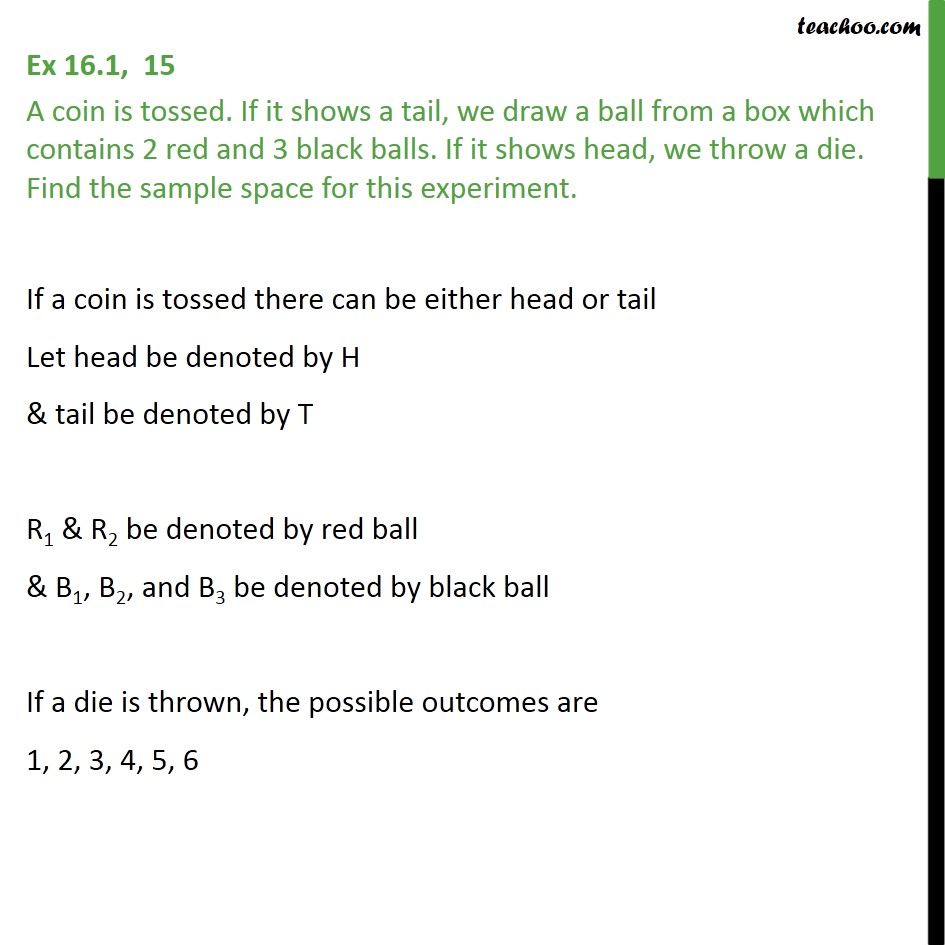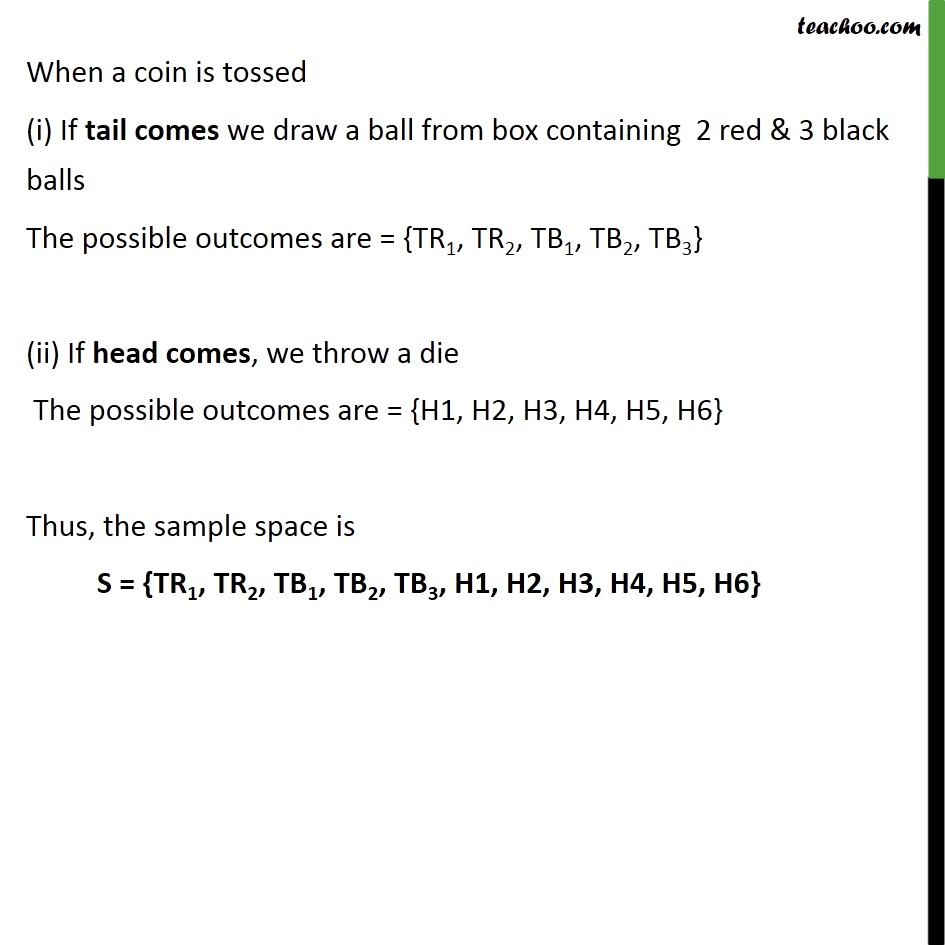Sample Space

Chapter 14 Class 11 Probability
Serial order wiseLearn in your speed, with individual attention - Teachoo Maths 1-on-1 Class

### Transcript

Question 15 A coin is tossed. If it shows a tail, we draw a ball from a box which contains 2 red and 3 black balls. If it shows head, we throw a die. Find the sample space for this experiment. If a coin is tossed there can be either head or tail Let head be denoted by H & tail be denoted by T R1 & R2 be denoted by red ball & B1, B2, and B3 be denoted by black ball If a die is thrown, the possible outcomes are 1, 2, 3, 4, 5, 6 When a coin is tossed (i) If tail comes we draw a ball from box containing 2 red & 3 black balls The possible outcomes are = {TR1, TR2, TB1, TB2, TB3} (ii) If head comes, we throw a die The possible outcomes are = {H1, H2, H3, H4, H5, H6} Thus, the sample space is S = {TR1, TR2, TB1, TB2, TB3, H1, H2, H3, H4, H5, H6}